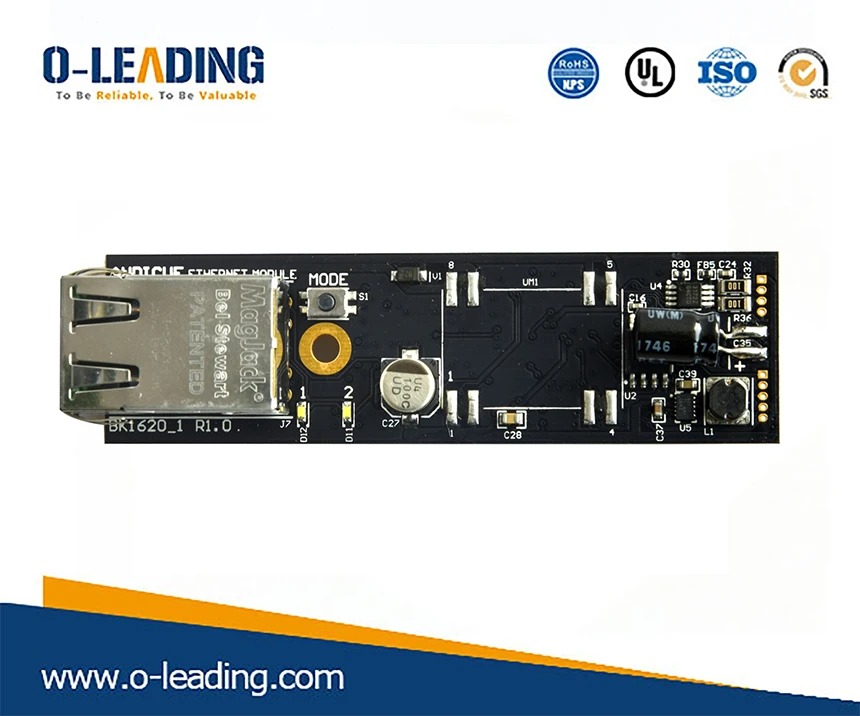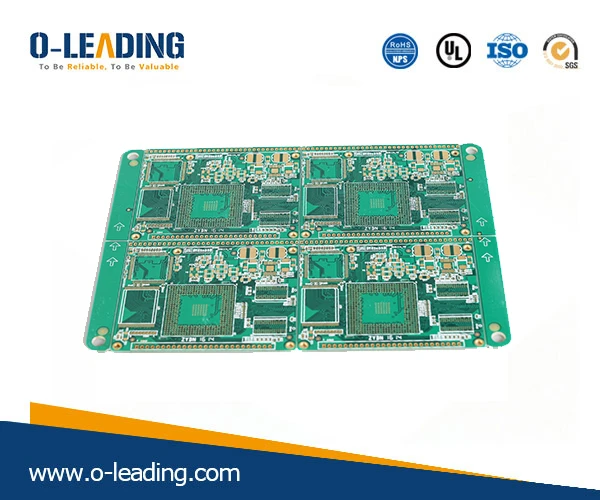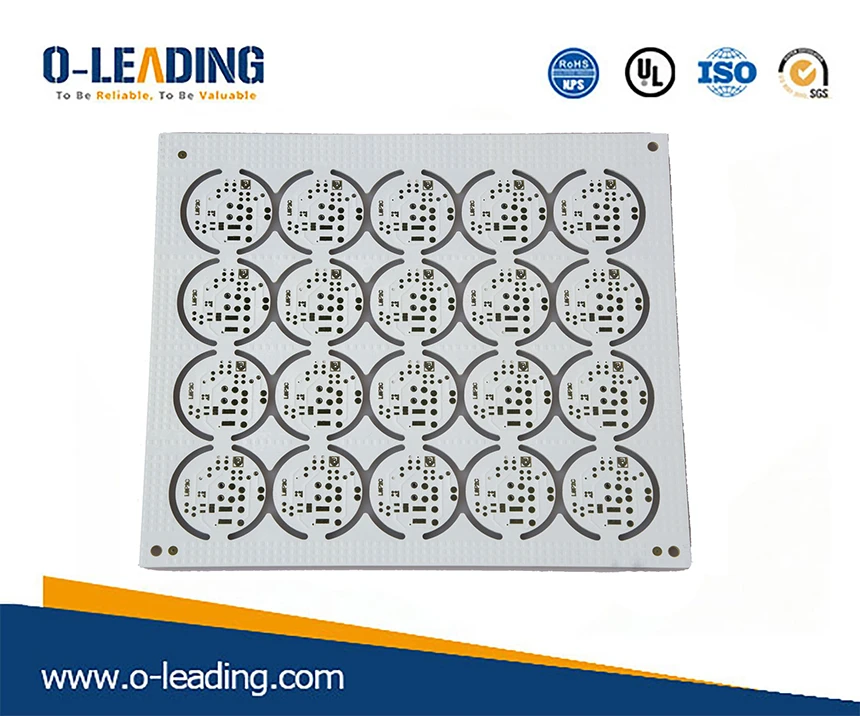Home > News > PCB News > Electromagnetic field solver classification
TEL: + 86-13428967267

FAX: + 86-4008892163-239121

+ 86-2028819702-239121

Email: sales@o-leading.comCertifications
New Products
Electronic album# Electromagnetic field solver classification

2019-07-24 10:23:47
In the design of electronic products, different electromagnetic field solvers may be used for different structures and requirements. The Field Solver is divided into dimensions: 2D, 2.5D, 3D; approximation types are divided into: static, quasi-static, TEM wave and full wave. RoHs Compliant manufacturer china.1. Quasi-electrostatic magnetic algorithm

It requires a three-dimensional structural model. The so-called "quasi-static" means that the system must support the existence of electrostatic fields and steady currents, which are expressed as the field of electrostatic field and static magnetic field. More precisely, the flux change rate or displacement current is very small, so in the Maxwell equations The partial derivatives of B and D versus time can be ignored, respectively, and the corresponding Maxwell equations are called quasi-static and quasi-static magnetism. The algorithm derived from this is called the quasi-electrostatic algorithm and the quasi-static magnetization algorithm. This type of algorithm is mainly used for EMC simulation in power frequency or low frequency power systems or electrical equipment. For example, the extraction of the distribution parameters between the bus bar and the cabinet can be completed by a quasi-electrostatic magnetic algorithm. For high-voltage insulation devices, it is obvious that a quasi-static approximation can be used, and for high-current devices such as converters, motors, transformers, etc., it is preferable to use a quasi-static magnetization algorithm. Multilayer PCB manufacturer in china.2, full wave electromagnetic algorithm

Simply put, it is an algorithm that solves the complete form of Maxwell's equations. The full-wave algorithm is divided into time domain and frequency domain algorithms. Finite Difference Method (FD), Finite Integral Method (FI), Transmission Line Matrix Method (TLM), Finite Element Method (FEM), Boundary Element Method (BEM), Moment Method (MoM), and Multilayer Fast Multipole Method ( MLFMM) is a full wave algorithm. All full-wave algorithms require volume mesh or face mesh segmentation of the simulation region. The first three methods (FD, FI, and TLM methods) are mainly time-domain explicit algorithms, and the sparse matrix, simulation time and memory are proportional to the grid number one time; the last four methods (FEM, BEM, MoM, and MLFMM) ) are frequency domain implicit algorithms. FEM is also a sparse matrix. The simulation time and memory are proportional to the square of the number of grids; while BEM and MoM are dense matrices, the time-to-memory ratio is the cube of the number of grids. FD, FI, TLM and FEM are suitable for any medium of any structure. BEM and MoM are suitable for any structure but uniform non-rotating medium distribution, while MLFMM is mainly suitable for metal convex structures, although MLFMM has super linear mesh convergence. That is known as the amount of NlogN calculation. 3D printer PCB supplier.3, 2D solver

The 2D solver is the simplest and most efficient, and is only suitable for simple applications. For example, a 2D static solver can extract the capacitance parameters of the cross-section of the on-chip interconnect. The 2D quasi-static solver can extract low-frequency RLGC parameters per unit length on the cross section of a uniform multi-conductor transmission line. The 2D full wave solver extracts full frequency RLGC parameters from the cross section of a uniform multiconductor transmission line. Typical 2D full wave calculation methods are: 2D boundary element method, 2D finite difference method, 2D finite element method.

4, 2.5D solver

The concept of 2.5D was put forward during the 1980s when Rautio was pursuing a Ph.D. at Syracuse University in the United States, where he worked as a planar MOM algorithm with the support of the GE Electronics Laboratory under Professor Roger. At that time, people only had the concept of 2D current (XY direction) and 3D electromagnetic field. People at GE Electronics Labs are concerned about currents, calling them 2D, while Professor Roger is concerned with electromagnetic fields and calls them 3D. Rautio and the two teams worked together. At the time, he was reading a book on fractal theory. The concept of subdimension was clearly defined in the book. So, Rautio was inspired to propose the concept of 2.5D, which is also the theory of fractal dimension. The first time it was used in the field of electromagnetic fields.

5, 3D solver

The 3D quasi-static solver is suitable for most 3D structures in chip-package-board systems, but it is effective for low frequencies, and the error of high frequency results is large. If the structure is large, the calculation time will be long and the memory consumption will be large.
The 3D full wave solver is the most accurate solver for the actual situation of the model. It can simulate all effects covered by RF, SI, PI, EMI, etc. Typical 3D full wave solvers are: boundary element method (Si9000), finite difference method (CST, Keysight EMpro/FDTD) and finite element method (Ansys) HFSS, Keysight Empro/ FEM).